﻿ 伪随机脉冲对卫星轨道拟合精度影响分析文章快速检索 高级检索
 大地测量与地球动力学2019, Vol. 39Issue (4): 387-391  DOI: 10.14075/j.jgg.2019.04.011### 引用本文YAN Zhichuang, LI Jing, LOU Nan. Influence of Pseudo-Stochastic Pulse on Satellite Orbit Fitting[J]. Journal of Geodesy and Geodynamics, 2019, 39(4): 387-391.### 第一作者简介

YAN Zhichuang, PhD, engineer, majors in precise orbit determination, algorithm research and software developing about GNSS & LEOs, E-mail:yanpipi2010@163.com.

### 文章历史

1. 西安测绘总站，西安市西影路36号，710054

1 卫星轨道拟合基本原理 1.1 卫星轨道拟合原理

 $\mathit{\boldsymbol{ \boldsymbol{\varPhi} }}\left( t \right) = \frac{{\partial \mathit{\boldsymbol{X}}}}{{\partial \mathit{\boldsymbol{X}}_0^{\rm{T}}}}$ (1)
 $\mathit{\boldsymbol{S}}\left( t \right) = \frac{{\partial \mathit{\boldsymbol{X}}}}{{\partial {\mathit{\boldsymbol{p}}^{\rm{T}}}}}$ (2)

 $\mathit{\boldsymbol{X}} = \mathit{\boldsymbol{\bar X}} + \frac{{\partial \mathit{\boldsymbol{\bar X}}}}{{\partial \mathit{\boldsymbol{X}}_0^{\rm{T}}}}\delta {\mathit{\boldsymbol{X}}_0} + \frac{{\partial \mathit{\boldsymbol{X}}}}{{\partial {\mathit{\boldsymbol{p}}^{\rm{T}}}}}\delta \mathit{\boldsymbol{p}} + \mathop \sum \limits_{i = 1}^n \frac{{\partial \mathit{\boldsymbol{X}}}}{{\partial \mathit{\boldsymbol{q}}_{{t_i}}^{\rm{T}}}}\delta {\mathit{\boldsymbol{q}}_{{t_i}}}$ (3)

1.2 伪随机脉冲基本模型

 ${\mathit{\boldsymbol{r}}_{{t_i}}} = r_{{t_i}}^ + = \mathit{\boldsymbol{r}}_{{t_i}}^ -$ (4)
 ${\mathit{\boldsymbol{v}}_{{t_i}}} = \mathit{\boldsymbol{v}}_{{t_i}}^ + = \mathit{\boldsymbol{v}}_{{t_i}}^ - + {\mathit{\boldsymbol{M}}_{{t_i}}}{\mathit{\boldsymbol{q}}_{{t_i}}}$ (5)

 $\frac{{\partial \mathit{\boldsymbol{\bar X}}}}{{\partial \mathit{\boldsymbol{q}}_{{t_i}}^{\rm{T}}}} = \left( {\begin{array}{*{20}{c}} \mathit{\boldsymbol{0}}\\ {{\mathit{\boldsymbol{M}}_{{t_i}}}} \end{array}} \right)$ (6)
2 卫星轨道拟合基本策略

3 低轨卫星轨道拟合精度分析表 1 R方向拟合轨道精度统计 Tab. 1 Orbit fitting precision in R direction表 2 T方向拟合轨道精度统计 Tab. 2 Orbit fitting precision in T direction表 3 N方向拟合轨道精度统计 Tab. 3 Orbit fitting precision in N direction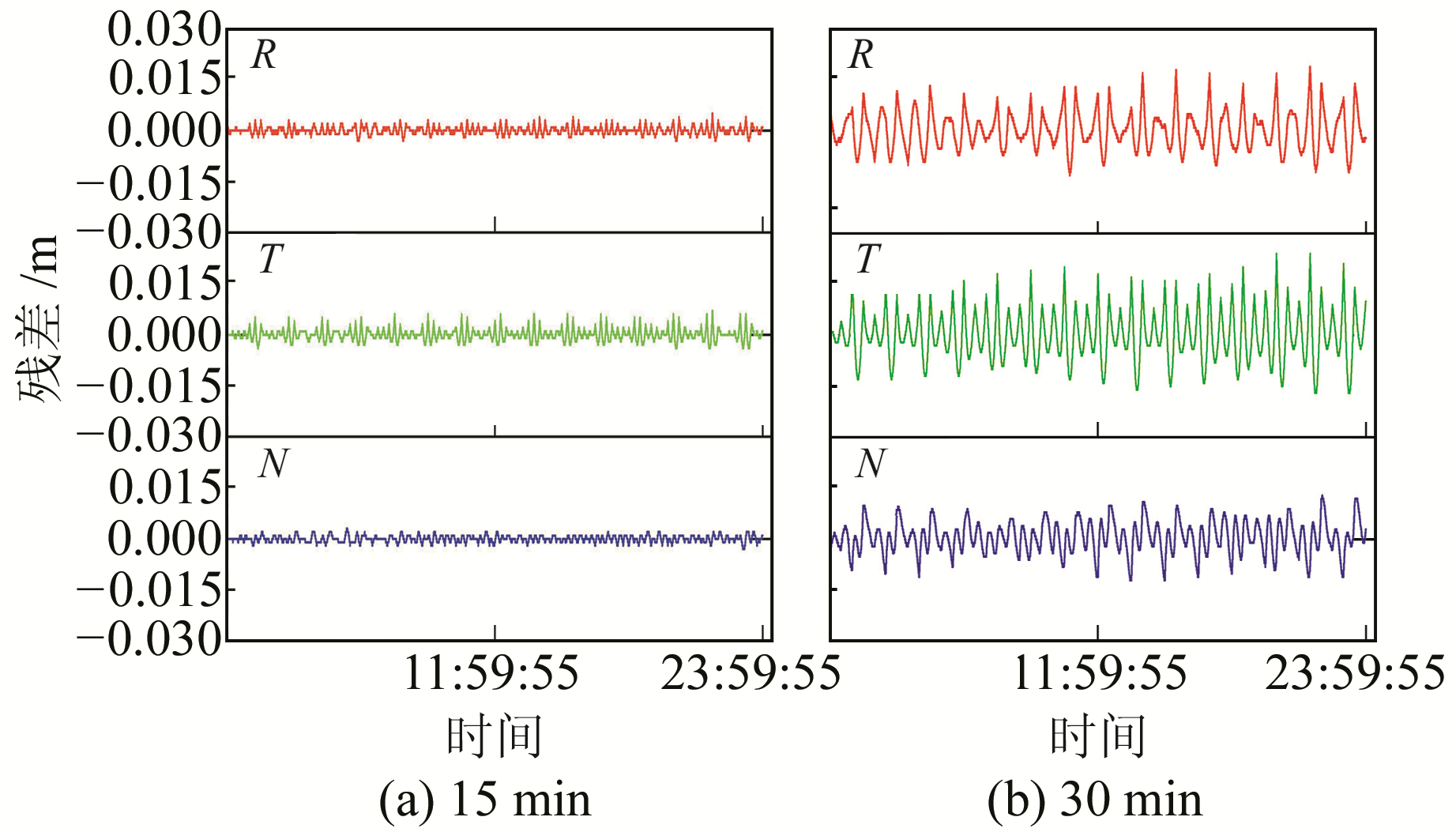图 1 年积日11 d拟合残差序列 Fig. 1 Fitting residual series for doy 11

4 GNSS卫星轨道拟合精度分析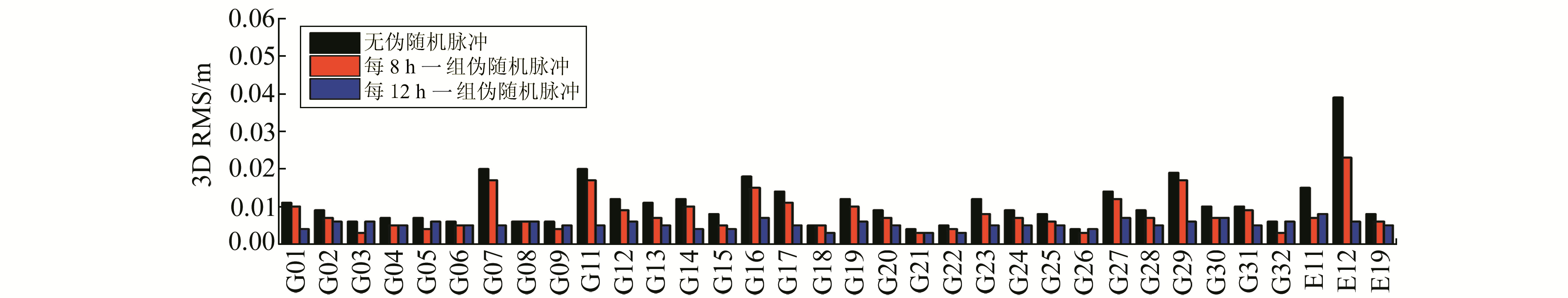图 2 方案1拟合轨道3D RMS比较(GPS+Galileo) Fig. 2 3D RMS of fitting orbit for scheme 1 (GPS+Galileo)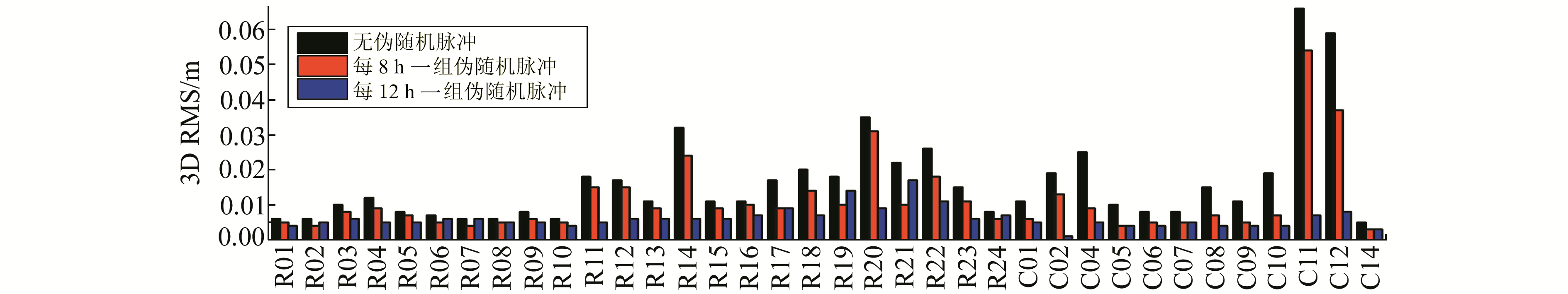图 3 方案1拟合轨道3D RMS比较(GLONASS+BDS) Fig. 3 3D RMS of fitting orbit for scheme 1 (GLONASS+BDS)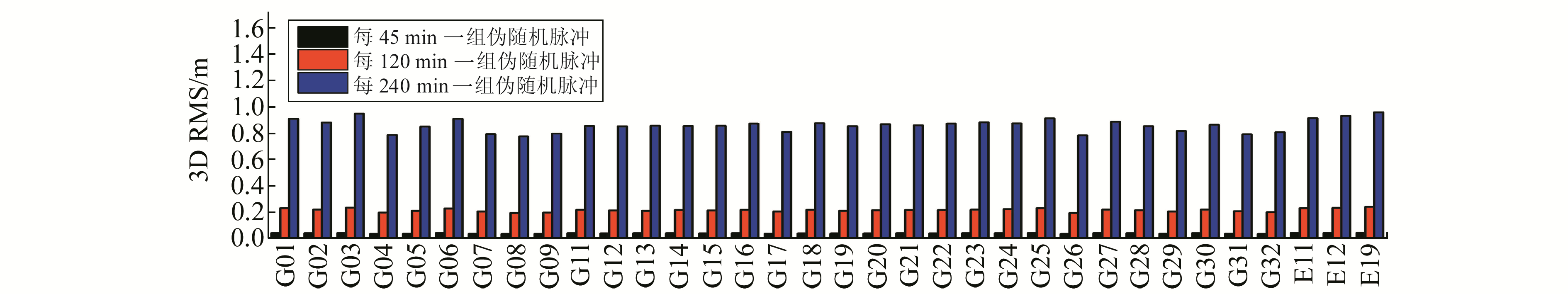图 4 方案2拟合轨道3D RMS比较(GPS+Galileo) Fig. 4 3D RMS of fitting orbit for scheme 2 (GPS+Galileo)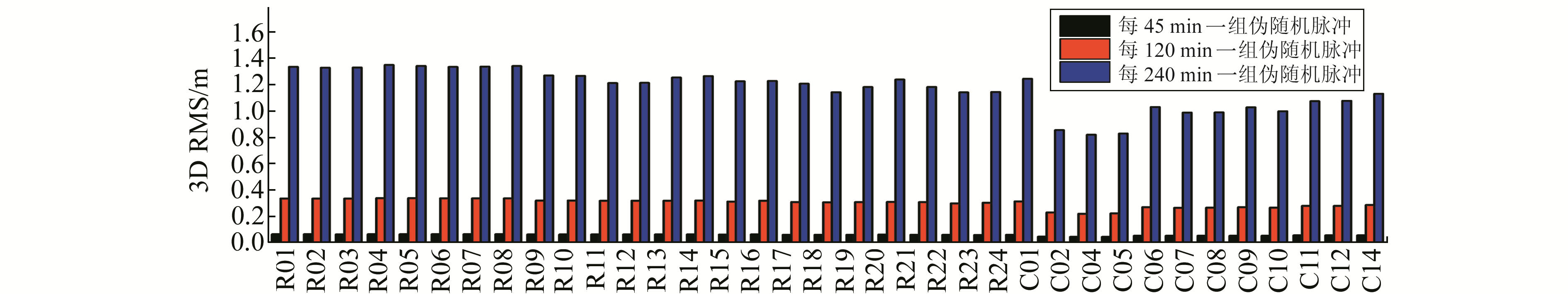图 5 方案2拟合轨道3D RMS比较(GLONASS+BDS) Fig. 5 3D RMS of fitting orbit for scheme 2 (GLONASS+BDS)

5 结语

1) 伪随机脉冲在吸收未建模摄动力方面具有良好的效果，低轨卫星轨道拟合中能很好地吸收大气阻力，拟合精度达mm级；GNSS卫星轨道拟合中能很好地吸收太阳光压摄动力，拟合精度达到cm级。

2) 使用伪随机脉冲吸收低轨卫星大气阻力和GNSS卫星太阳光压摄动时，设置周期越短，吸收效果越好，拟合精度越高，反之拟合精度越低。但伪随机脉冲次数越多，解算待估参数越多，解算效率会明显降低。

3) 尽管伪随机脉冲能够有效吸收GNSS卫星太阳光压摄动影响，但进一步提高轨道拟合精度需要与ECOM太阳光压模型组合使用，每天设置1组伪随机脉冲即可保证拟合精度达到mm级，次数设置过多，拟合精度反而会下降。

4) 使用伪随机脉冲吸收低轨卫星和GNSS卫星未模型化的摄动力，本质上是以牺牲卫星速度的连续性为代价，而GNSS精密星历通常应用在地面精密定位等场合，一般不需要卫星的速度，只要能保证卫星轨道的高精度，卫星速度是否连续不影响地面用户的使用。

5) 在设置GRACE卫星伪随机脉冲间隔时直接使用了10 min、15 min和30 min固定间隔，主要考虑到GRACE卫星运行周期约为90 min，伪随机脉冲设置间隔不能超过其周期，同时也是一种经验做法，但脉冲时间间隔与拟合精度之间的理论推导及定量关系还有待进一步研究及完善。

  张睿, 杨元喜. BDS/GPS联合定轨的贡献分析[J]. 武汉大学学报:信息科学版, 2017, 42(5): 600-608 (Zhang Rui, Yang Yuanxi. Contribution Analysis of BDS/GPS Combined Orbit Determination[J]. Geomatics and Information Science of Wuhan University, 2017, 42(5): 600-608) (0)  匡翠林.利用GPS非差数据精密确定低轨卫星轨道的理论及方法研究[D].武汉: 武汉大学, 2008 (Kuang Cuilin. Research on Precise Orbit Determination Theory and Methods of Lower Earth Orbit Satellites Using Zero-Difference GPS Data[D]. Wuhan: Wuhan University, 2008) (0)  Montenbruck O, Gill E, Kroes R. Rapid Orbit Determination of LEO Satellites Using IGS Clock and Ephemeris Products[J]. GPS Solutions, 2005, 9(3): 226-235 DOI:10.1007/s10291-005-0131-0 (0)  Schrama E J O, Visser P N A M. Accuracy Assessment of the Monthly GRACE Geoids Based upon a Simulation[J]. Journal of Geodesy, 2007, 81(1): 67-80 (0)  Pail R. CHAMP-, GRACE-, GOCE-Satellite Projects[A]//Grafarend E. Encyclopedia of Geodesy[M]. Cham: Springer, 2015 (0)  Jäggi A, Beutler G, Mervart L. GRACE Gravity Field Determination Using the Celestial Mechanics Approach-First Results[A]//Mertikas S P. Gravity, Geoid and Earth Observation[M]. Heidelberg: Springer, 2010 (0)  陈俊平, 王解先. GPS定轨中的太阳辐射压模型[J]. 天文学报, 2006, 47(3): 310-319 (Chen Junping, Wang Jiexian. Solar Radiation Pressure Models for the GPS Satellites[J]. Acta Astronomica Sinica, 2006, 47(3): 310-319 DOI:10.3321/j.issn:0001-5245.2006.03.009) (0)  Beutler G, Jäggi A, Hugentobler U, et al. Efficient Satellite Orbit Modelling Using Pseudo-Stochastic Parameters[J]. Journal of Geodesy, 2006, 80(7): 353-372 DOI:10.1007/s00190-006-0072-6 (0)  Guo J L, Hu M, Zhao Q L, et al. Reducing Influence of Gravity Model Error in Precise Orbit Determination of Low Earth Orbit Satellites[J]. Geo-Spatial Information Science, 2007, 10(2): 105-110 DOI:10.1007/s11806-007-0055-7 (0)  韩保民, 朱秀英. 伪随机脉冲估计及其在简化动力学定轨中的应用[J]. 武汉大学学报:信息科学版, 2007, 32(5): 466-469 (Han Baomin, Zhu Xiuying. Estimation of Pseudo-Stochastic Pulses and Their Applications in Reduced-Dynamic Orbit Determination[J]. Geomatics and Information Science of Wuhan University, 2007, 32(5): 466-469) (0)  赵春梅, 程鹏飞. 基于伪随机脉冲估计的简化动力学卫星定轨方法[J]. 宇航学报, 2011, 32(4): 762-766 (Zhao Chunmei, Cheng Pengfei. Reduced-Dynamics Satellite Orbit Determination Based on Pseudo-Stochastic Pulse Estimation[J]. Journal of Astronautics, 2011, 32(4): 762-766 DOI:10.3873/j.issn.1000-1328.2011.04.009) (0)  刘伟平, 郝金明, 田英国, 等. 一种伪随机脉冲的快速参数估计方法[J]. 武汉大学学报:信息科学版, 2015, 40(11): 1487-1492 (Liu Weiping, Hao Jinming, Tian Yingguo, et al. Fast Parameter Estimation for Pseudo-Stochastic Pulse[J]. Geomatics and Information Science of Wuhan University, 2015, 40(11): 1487-1492) (0)  田英国, 郝金明, 谢建涛, 等. SWARM卫星星载GPS精密定轨方法及精度分析[J]. 测绘科学技术学报, 2016, 33(5): 452-457 (Tian Yingguo, Hao Jinming, Xie Jiantao, et al. SWARM Precise Orbit Determination and Accuracy Analysis Using Onboard GPS Data[J]. Journal of Geomatics Science and Technology, 2016, 33(5): 452-457) (0)  闫志闯. GRACE卫星精密轨道确定与一步法恢复地球重力场[D].郑州: 信息工程大学, 2015 (Yan Zhichuang. Precise Orbit Determination and the Earth Gravity Field Recovery by One Step Method for GRACE[D]. Zhengzhou: Information Engineering University, 2015) http://cdmd.cnki.com.cn/Article/CDMD-90005-1016058452.htm (0)
Influence of Pseudo-Stochastic Pulse on Satellite Orbit Fitting
YAN Zhichuang1LI Jing1     LOU Nan1
1. Xi'an Technical Division of Surveying and Mapping, 36 Xiying Road, Xi'an 710054, China
Abstract: We study the influence of pseudo-stochastic pulse in the absorption of low earth orbit (LEO) satellites and the unmodeled perturbation forces of four navigation systems. We also analyze the influence of accuracy on fitting orbit. The results show that pseudo-stochastic pulse can effectively absorb the unmodeled perturbation forces of LEO and GNSS satellites. For LEO satellites, pseudo-stochastic pulse can simulate the influence of atmospheric resistance on orbit, and achieve millimeter level fitting precision. For GNSS satellites, pseudo-stochastic pulse can only absorb sun light pressure perturbation to some extent; however, combined with the ECOM sun light model it can fully absorb perturbation forces unabsorbed by the ECOM model and achieve millimeter level fitting precision, requiring setting up a set of pseudo-stochastic pulse parameters every day.
Key words: orbit fitting; pseudo-stochastic pulse; SPODAGRS